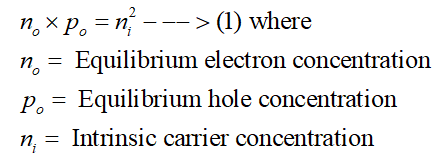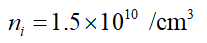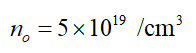# At 300 K, the equilibrium electron concentration in a doped silicon sample is 5x1019/cm3. Determine the equilibrium hole concentration.

Question
1 views

At 300 K, the equilibrium electron concentration in a doped silicon sample is 5x1019/cm3. Determine the equilibrium hole concentration.

check_circle

star
star
star
star
star
1 Rating
Step 1

According to the law of mass action for semiconductors, the product of the equilibrium electron and hole concentration is equal to the square of the intrinsic carrier concentration. This can be expressed asStep 2

For Silicon (Si), the intrinsic carrier concentration at 300 K can be expressed asStep 3

In this case, for the doped Silicon substrate, the value of the equilibrium electron concentration is given as...

### Want to see the full answer?

See Solution

#### Want to see this answer and more?

Solutions are written by subject experts who are available 24/7. Questions are typically answered within 1 hour.*

See Solution
*Response times may vary by subject and question.
Tagged in